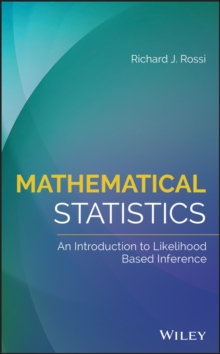Supporting your high street Find out how »
• My AccountMathematical Statistics : An Introduction to Likelihood Based Inference Hardback

Description

Presents a unified approach to parametric estimation, confidence intervals, hypothesis testing, and statistical modeling, which are uniquely based on the likelihood function This book addresses mathematical statistics for upper-undergraduates and first year graduate students, tying chapters on estimation, confidence intervals, hypothesis testing, and statistical models together to present a unifying focus on the likelihood function.

It also emphasizes the important ideas in statistical modeling, such as sufficiency, exponential family distributions, and large sample properties.

Mathematical Statistics: An Introduction to Likelihood Based Inference makes advanced topics accessible and understandable and covers many topics in more depth than typical mathematical statistics textbooks.

It includes numerous examples, case studies, a large number of exercises ranging from drill and skill to extremely difficult problems, and many of the important theorems of mathematical statistics along with their proofs. In addition to the connected chapters mentioned above, Mathematical Statistics covers likelihood-based estimation, with emphasis on multidimensional parameter spaces and range dependent support.

It also includes a chapter on confidence intervals, which contains examples of exact confidence intervals along with the standard large sample confidence intervals based on the MLE's and bootstrap confidence intervals.

There's also a chapter on parametric statistical models featuring sections on non-iid observations, linear regression, logistic regression, Poisson regression, and linear models. Prepares students with the tools needed to be successful in their future work in statistics data scienceIncludes practical case studies including real-life data collected from Yellowstone National Park, the Donner party, and the Titanic voyageEmphasizes the important ideas to statistical modeling, such as sufficiency, exponential family distributions, and large sample propertiesIncludes sections on Bayesian estimation and credible intervalsFeatures examples, problems, and solutions Mathematical Statistics: An Introduction to Likelihood Based Inference is an ideal textbook for upper-undergraduate and graduate courses in probability, mathematical statistics, and/or statistical inference.

Information

• Format: Hardback
• Pages: 448 pages
• Publisher: John Wiley & Sons Inc
• Publication Date:
• Category: Calculus & mathematical analysis
• ISBN: 9781118771044

£94.95

£79.65

on all orders

Pick up orders

from local bookshops

£118.00

£104.39

£127.00

£112.65